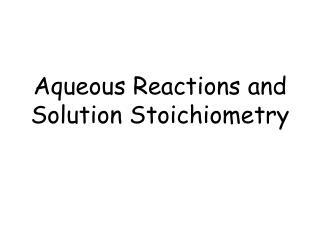DownloadDownload PresentationAqueous Reactions and Solution Stoichiometry

# Aqueous Reactions and Solution Stoichiometry

Télécharger la présentation## Aqueous Reactions and Solution Stoichiometry

- - - - - - - - - - - - - - - - - - - - - - - - - - - E N D - - - - - - - - - - - - - - - - - - - - - - - - - - -
##### Presentation Transcript

1. Aqueous Reactions and Solution Stoichiometry

2. Aqueous Solutions • Aqueous solutions are solutions in which water does the dissolving. • Solute – material that is dissolved • Solvent – the material doing the dissolving

3. Properties of Aqueous Solutions • Electrolytic properties • Ability to conduct an electric current • Ionic compounds are electrolytic solutions • Acids and bases are electrolytic

4. Electrolytic Solutions • Ionic Compounds • Dissociate in water into their component ions • NaCl (s) → Na+ (aq) + Cl- (aq)

5. Try These! • What dissolved species are present in a solution of: • KCN → K+ (aq) + CN- (aq) • NaClO4→ Na+(aq) + ClO4- (aq)

6. Molecular Compounds • Molecular compounds are not made up of ions and therefore do not ionize in water. • They are non-conductors of electricity.

7. Strong vs. Weak Electrolytes • Strong electrolytes – exist completely as ions in solution • HCl → H+(aq) + Cl- (aq) • Weak electrolytes – only a small fraction of the compound exists as ions in solution. • CH3COOH → CH3COO-(aq) + H+(aq)

8. Electrolytic solutions • CH3COOH is very soluble, but a weak electrolyte. • Ba(OH)2 is not very soluble, but is a strong electrolyte.

9. Ionic solution Molecular solution

10. Solubility and Precipitation Reactions • These are reactions that result in the formation of an insoluble product. • A precipitate is a insoluble solid formed by a chemical reaction in a solution. • 2KI (aq) + Pb(NO3)2(aq)→ PbI2(aq) + 2KNO3(aq)

11. Double Replacement Reactions(Metathesis Reactions) • These reactions can go to completion (are not reversible) if the following are produced: • Precipitate is formed (insoluble solid is formed) • Gas is formed • Formation of a primarily molecular species • Ca(CH3COO)2 + 2HCl → CaCl2 + 2CH3COOH • 2Na3PO4 + 3H2SO4 → Na2SO4 + 2H3PO4 • HCl + NaOH → NaCl + HOH

12. Acid Base Neutralization Reactions • Acids react with bases to produce water and a salt. • Acid + base → salt + water Strong acids are written in ionic form and weak acids are written in molecular form. Common strong acids are: HClO4, HClO3, HCl, HBr, HNO3 and H2SO4 - memorize these!

13. Total Ionic Equations • Formulas of the reactants and products are written to show the predominant form of each substance as it exists in an aqueous solution. • Soluble salts, strong acids and strong bases are written as separated ions. • Insoluble salts, suspensions, solids, weak acids and bases, gases and water are written as individual molecules.

14. Total Ionic Equations • Example: Cd2+(aq) + 2NO3-(aq) + 2Na+(aq) + S2-(aq)→ CdS(s) + 2Na+(aq) + 2NO3-(aq)

15. Net Ionic Equations • Cancel out all spectator ions that do not participate in the reaction. • The remaining equation is the net ionic equation.

16. Redox Equation Balancing and Reactions • Loss of electrons is Oxidation • Gain of electrons is Reduction • LEO the Lion goes GER!

17. Redox Equations • Assign the oxidation states of all elements in the compounds in the equation. • Identify which ones undergo oxidation and which ones undergo reduction. (Look for changes in oxidation from one side of the arrow to the other.) • Use this to balance the overall equation.

18. Molarity • Molarity is the concentration of a substance in solution. moles of solute Molarity = ------------------------------- liters of solution

19. Molarity of Electrolytes • When an ionic substance dissolves in water, the relative concentrations in the solution depend on the chemical formula of the compound. • A 1.0 M solution of NaCl is 1.0 M of Na+ ions and 1.0 M Cl- ions. • A 1.0 M solution of Na2SO4 is 2.0 M of Na+ ions and 1.0 M of SO42- ions.

20. Dilution • Dilutions of solutions are frequently used when starting from a concentrated stock solution, or a concentrated solution of an acid, such as HCl. • M1V1 = M2V2

21. Titrations • This determines the concentration of an unknown solution using a known standard solution. • Acid base indicators are frequently used to determine the end point of a titration.